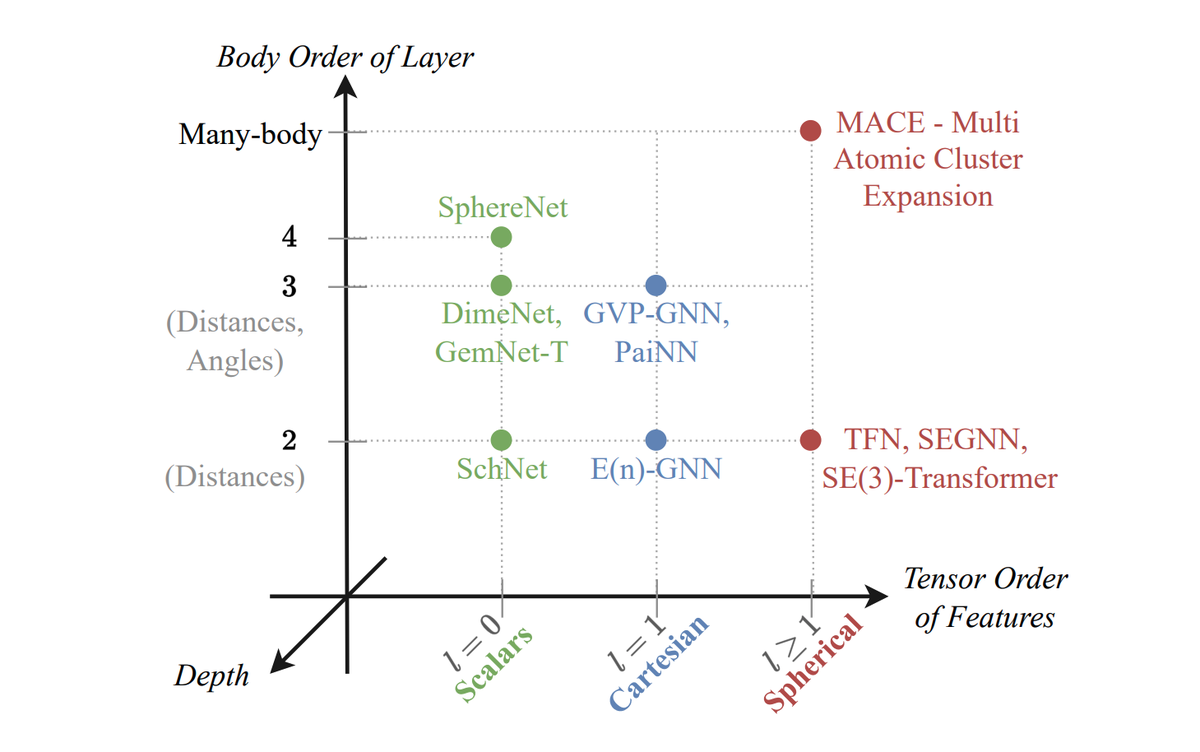# On the Expressive Power of Geometric Graph Neural Networks### Abstract

The expressive power of Graph Neural Networks (GNNs) has been studied extensively through the Weisfeiler-Leman (WL) graph isomorphism test. However, standard GNNs and the WL framework are inapplicable for geometric graphs embedded in Euclidean space, such as biomolecules, materials, and other physical systems. In this work, we propose a geometric version of the WL test (GWL) for discriminating geometric graphs while respecting the underlying physical symmetries: permutations, rotation, reflection, and translation. We use GWL to characterise the expressive power of geometric GNNs that are invariant or equivariant to physical symmetries in terms of distinguishing geometric graphs. GWL unpacks how key design choices influence geometric GNN expressivity: (1) Invariant layers have limited expressivity as they cannot distinguish one-hop identical geometric graphs; (2) Equivariant layers distinguish a larger class of graphs by propagating geometric information beyond local neighbourhoods; (3) Higher order tensors and scalarisation enable maximally powerful geometric GNNs; and (4) GWL’s discrimination-based perspective is equivalent to universal approximation. Synthetic experiments supplementing our results are available at https://github.com/chaitjo/geometric-gnn-dojo

Publication
International Conference on Machine Learning (ICML)